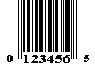## UPC-E Specification

It is intended to be used on packaging which would be otherwise too small to use one of the other versions. The code is smaller because it drops out zeros which would otherwise occur in a symbol. For example, the code 59300-00066 would be encoded as 593663. The last digit (3 in the example) indicates the type of compression. Guard bars precede and follow the data (no middle guard bars). The digits are coded following the parity pattern EVEN, EVEN, ODD, ODD, EVEN, ODD. The data is enclosed between two left-hand guard bars and three right-hand guard bars. The six digit number is always preceeded by a 0 and followed by the check digit. The way the check digit is computed is by expanding the type E to a type A, then doing the regular check.UPC-E (The zero-suppression version)is similar to the portion of Version A to the left of the center except for the following:

 It has a right guard pattern which is coded 010101. Three of the characters are coded in odd parity and three are in even parity.

Character encodation for UPC-E is given in the following table.

### Even Parity

0 0001101 0100111
1 0011001 0110011
2 0010011 0011011
3 0111101 0100001
4 0100011 0011101
5 0110001 0111001
6 0101111 0000101
7 0111011 0010001
8 0110111 0001001
9 0001011 0010111

Only number system "0" can be represented by zero suppression symbols.

The coding of the zero-suppression version is compressed into six characters of varying parity. The determination of whether a character's parity is even or odd is per the following table.

## 6

0 E E E O O O
1 E E O E O O
2 E E O O E O
3 E E O O O E
4 E O E E O O
5 E O O E E O
6 E O O O E E
7 E O E O E O
8 E O E O O E
9 E O O E O E

### Check Digit Calculation

```Step 1: Designate the least significant digit postion as odd.

9 0 1 2 5 8
E O E O E O

Step 2: Sum all the digits in the odd (O) positions

0 + 2 + 8 = 10

Step 3: Multiply the result by 3

10 * 3 = 30

Step 4: Sum all the digits in the even (E) positions.

9 + 1 + 5 = 15

Step 5: Add the result of Step 2 with the result of Step 4.

30 + 15 = 45

Step 6: Subtract from the next higher multiple of 10..

50 - 45 = 5.

In this example, the check digit is 5.```